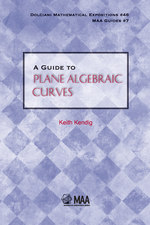Have library access? Log in through your library# A Guide to Plane Algebraic Curves

Keith Kendig
Volume: 46
Copyright Date: 2011
Edition: 1
Pages: 210
https://www.jstor.org/stable/10.4169/j.ctt6wpwvv

## Table of Contents

1. Front Matter
(pp. I-VI)
2. Preface
(pp. VII-XII)
Keith Kendig
3. Table of Contents
(pp. XIII-XVI)
4. CHAPTER 1 A Gallery of Algebraic Curves
(pp. 1-28)

A great way to learn new mathematics is to work with examples. That’s how we start. This chapter consists mostly of examples of algebraic curves in the real plane. A plane algebraic curve is defined to be the locus, or set of zeros, of a polynomial in two Cartesian variables with real coefficients. This may sound pretty special, but a surprisingly large number of familiar curves are exactly of this type. For example, many polar coordinate curves — lemniscates, limaçons, all sorts of roses, folia, conchoids—are algebraic, as are many curves defined parametrically, such as Lissajous figures and the...

5. CHAPTER 2 Points at Infinity
(pp. 29-44)

The examples in the last chapter reveal a wide range of behavior of algebraic curves in the real plane.

Some are bounded, others are not.

Some form one piece — that is, they’re connected, having just one topological component — while others are not.

For a curve having two or more topological components, there can be a mixture of bounded and unbounded components. For example, in Figure 1.10 on p. 19, all components are bounded. The middle right graph of Figure 1.3 on p. 8 has a bounded and an unbounded component together. In a hyperbola, both branches are unbounded....

6. CHAPTER 3 From Real to Complex
(pp. 45-74)

We have hinted several times at a multiplication theorem for two intersecting algebraic curves, and it is now time to make a promise. In this chapter, we state such a theorem and sketch its proof.

Looking at the parabolay=x² and the liney= 1 suggests what is needed to accomplish our aim. These curves have degree two and one, and intersect in 2.1 points. As we parallel-translate the line downward, the two points of intersection approach each other, and when the line coincides with the x-axis, the points have coalesced, “piling up on each other” at...

7. CHAPTER 4 Topology of Algebraic Curves in \${{\mathbb{P}}^{2}}(\mathbb{C})\$
(pp. 75-92)

The gallery of real curves in Chapter 1 presented a wide range of behavior. It was so wide, we were led to ask “Where are the nice theorems?” We’ve already seen how broadening curves’ living space to\${{\mathbb{P}}^{2}}(\mathbb{C})\$can lead to more unified results, Bézout’s theorem in Chapter 3 being a prime example. But what about those real curves we met in Chapter 1 having more than one connected component? Or ones having mixed dimensions? Does working in\${{\mathbb{P}}^{2}}(\mathbb{C})\$perform its magic for cases like this?

Yes. In this chapter we’ll see that individual curves in\${{\mathbb{P}}^{2}}(\mathbb{C})\$are generally much...

8. CHAPTER 5 Singularities
(pp. 93-142)

We have met curves that aren’t everywhere smooth. For example in\${{\mathbb{R}}^{2}}\$, the curvey² =x³ has a cusp at the origin, and in a neighborhood of the origin the alpha curvey² =x²(x+ 1)is ×-shaped. Each of these points is asingularityof the curve. The term “singular” connotes exceptional or rare. Within any particular complex affine or projective curve, singular points are indeed rare because there are only finitely many of them among the infinitely many points of the curve. A curve having no singularities is callednonsingular.

Singular points are rare in yet...

9. CHAPTER 6 The Big Three: C, K, S
(pp. 143-184)

There are three central players in our subject. Although to the unsuspecting they may appear quite different, the unreasonable truth is that they’re one and the same, each in different clothing. Without all the proper definitions just yet, they are

C, an irreducible curve in\${{\mathbb{P}}^{2}}(\mathbb{C})\$;

K, a field of transcendence degree 1 over\${\mathbb {C}}\$;

S, a compact Riemann surface which, for the moment, can be thought of as a nonsingular curve in\${{\mathbb{P}}^{3}}(\mathbb{C})\$.

Each of these three has a notion of equivalence, and there are equivalences from any one to any other.

Uniting the apparently dissimilar...

10. Bibliography
(pp. 185-188)
11. Index
(pp. 189-192)
12. About the Author
(pp. 193-193)